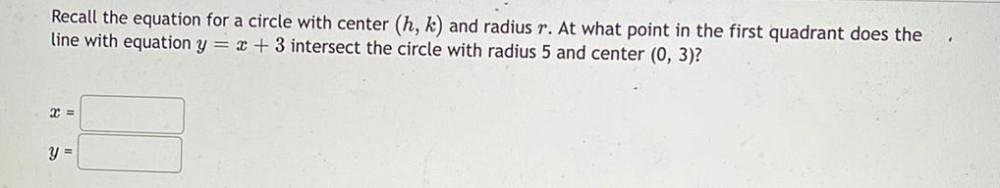Question:

# Recall the equation for a circle with center (h, k) and

Last updated: 7/26/2022Recall the equation for a circle with center (h, k) and radius r. At what point in the first quadrant does the line with equation y = x + 3 intersect the circle with radius 5 and center (0, 3)? x = _________ y = _________